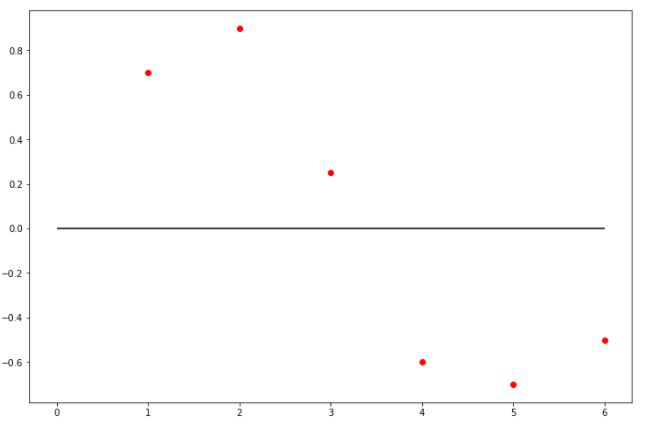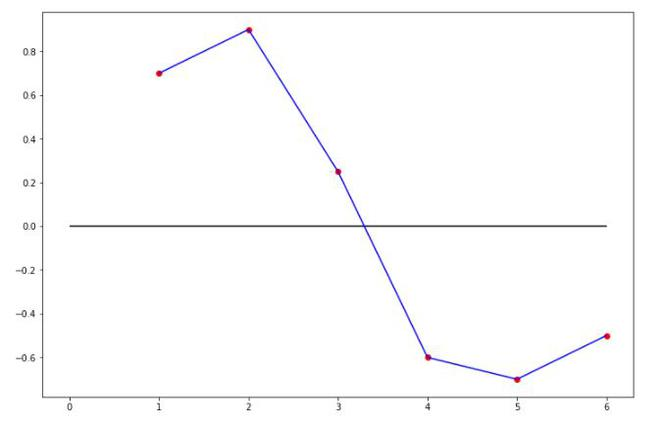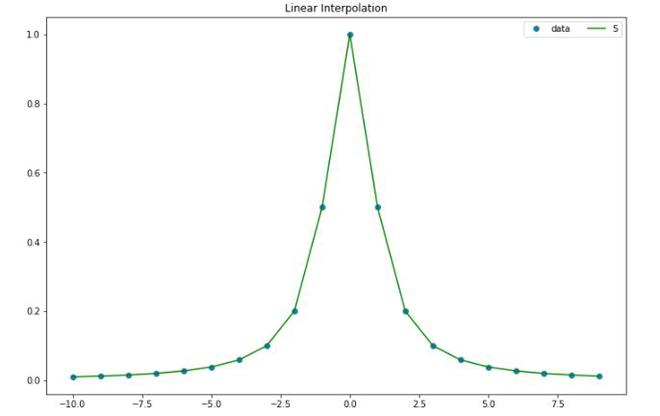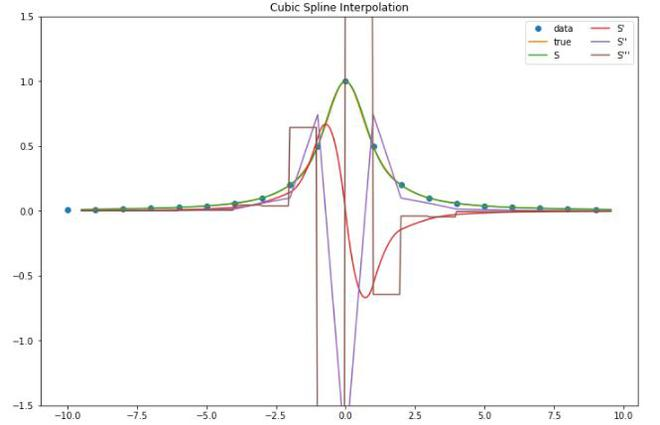GeeksforGeeks App
Open AppBrowser
Continue

# Cubic spline Interpolation

### Interpolation:

We estimate f(x) for arbitrary x, by drawing a smooth curve through the xi. If the desired x is between the largest and smallest of the xi then it is called interpolation, otherwise, it is called Extrapolation.Random points

#### Linear Interpolation:

Linear Interpolation is a way of curve fitting the points by using linear polynomial such as the equation of the line. This is just similar to joining points by drawing a line b/w the two points in the dataset.Linear Interpolation

#### Polynomial Interpolation:

Polynomial Interpolation is the way of fitting the curve by creating a higher degree polynomial to join those points.

#### Spline Interpolation:

Spline interpolation similar to the Polynomial interpolation x’ uses low-degree polynomials in each of the intervals and chooses the polynomial pieces such that they fit smoothly together. The resulting function is called a spline.

### Cubic Spline Interpolation

Cubic spline interpolation is a way of finding a curve that connects data points with a degree of three or less. Splines are polynomial that are smooth and continuous across a given plot and also continuous first and second derivatives where they join.

We take a set of points [xi, yi] for i = 0, 1, …, n for the function y = f(x). The cubic spline interpolation is a piecewise continuous curve, passing through each of the values in the table.

• Following are the conditions for the spline of degree K=3:
• The domain of s is in intervals of [a, b].
• S, S’, S” are all continuous function on [a, b].Here Si(x) is the cubic polynomial that will be used on the subinterval [xi, xi+1]

The main factor about spline is that it combines different polynomials and not use a single polynomial of degree n to fit all the points at once, it avoids high degree polynomials and thereby the potential problem of overfitting. These low-degree polynomials need to be such that the spline they form is not only continuous but also smooth.

But for the spline to be smooth and continuous, the two consecutive polynomials and Si (x) and Si+1 (x) must join at xiOr, Si (x) must be passed through two end-points:Assume, S” (x) = Mi (i= 0,1,2, …, n). Since S(x) is cubic polynomial, so S” (x) is the linear polynomial in [xi, xi+1], then S”’ (x) will be:By applying the Taylor series:Let, x = xi+1:Similarly, we apply above equation b/w range [xi-1, xi]:Let hi =xi – xi-1Now, we have n-1 equations, but have n+1 variables i.e M0, M1, M2,…Mn-1, Mn. Therefore, we need to get 2 more equation. For that, we will be using additional boundary conditions.

Let’s consider that we know S’ (x0) = f0‘ and S’ (xn) = fn, especially if S’ (x0) and S’ (xn) both are 0. This is called the clamped boundary condition.Similarly, for MnorCombining the above equation in to the matrix form, we get the following matrix:### Implementation

• We will be using the Scipy to perform the linear spline interpolation. We will be using Cubic Spline and interp1d function of scipy to perform interpolation of function f(x) =1/(1+x^2).

## Python3

 #importsimport matplotlib.pyplot as pltimport numpy as npfrom scipy.interpolate import CubicSpline, interp1dplt.rcParams['figure.figsize'] =(12,8)  x = np.arange(-10,10)y = 1/(1+x**2)# apply cubic spline interpolationcs = CubicSpline(x, y)# Apply Linear interpolationlinear_int = interp1d(x,y)  xs = np.arange(-10, 10)ys = linear_int(xs)  # plot linear interpolationplt.plot(x, y, 'o', label='data')plt.plot(xs,ys,  label="S", color='green')plt.legend(loc='upper right', ncol=2)plt.title('Linear Interpolation')plt.show()  # plot cubic spline interpolationplt.plot(x, y, 'o', label='data')plt.plot(xs, 1/(1+(xs**2)), label='true')plt.plot(xs, cs(xs), label="S")plt.plot(xs, cs(xs, 1), label="S'")plt.plot(xs, cs(xs, 2), label="S''")plt.plot(xs, cs(xs, 3), label="S'''")plt.ylim(-1.5, 1.5)plt.legend(loc='upper right', ncol=2)plt.title('Cubic Spline Interpolation')plt.show()Linear InterpolationCubic Spline interpolation

### References:

My Personal Notes arrow_drop_up